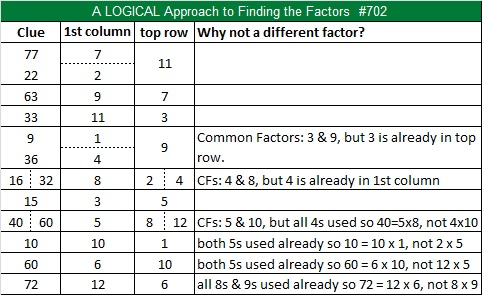# 702 A Couple of Christmas Factor Trees

Since the sum of its digits equals nine, 702 is divisible by nine.

• 702 is a composite number.
• Prime factorization: 702 = 2 x 3 x 3 x 3 x 13, which can be written 702 = 2 x (3^3) x 13
• The exponents in the prime factorization are 1, 3, and 1. Adding one to each and multiplying we get (1 + 1)(3 + 1)(1 + 1) = 2 x 4 x 2 = 16. Therefore 702 has exactly 16 factors.
• Factors of 702: 1, 2, 3, 6, 9, 13, 18, 26, 27, 39, 54, 78, 117, 234, 351, 702
• Factor pairs: 702 = 1 x 702, 2 x 351, 3 x 234, 6 x 117, 9 x 78, 13 x 54, 18 x 39, or 26 x 27
• Taking the factor pair with the largest square number factor, we get √702 = (√9)(√78) = 3√78 ≈ 26.49528.702 is the product of consecutive integers: 26 x 27 = 702. Numbers that can be expressed as such products are known as Pronic numbers.

It seems only natural to make factor trees based on those two multiplication facts:Today’s Find the Factors puzzle also looks like a couple of small Christmas trees.Print the puzzles or type the solution on this excel file: 12 Factors 2015-11-30

———————————————————————————

Here are more facts about the number 702:

It is the sum of consecutive prime numbers 349 and 353.

It is also the sum of the seventeen prime numbers from 7 to 73.

And because 13 is one of its factors, 702 is the hypotenuse of Pythagorean triple 270-648-702. Notice that the short leg is a permutation of 702.

———————————————————————————This site uses Akismet to reduce spam. Learn how your comment data is processed.